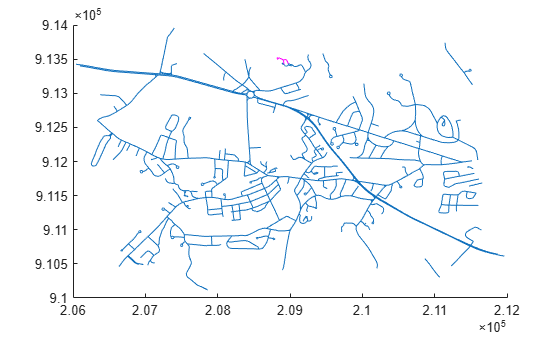# extractfield

Field values from structure array

## Syntax

``a = extractfield(S,name)``

## Description

example

````a = extractfield(S,name)` returns the field values specified by the field `name` of structure `S`.```

## Examples

collapse all

```roads = shaperead('concord_roads.shp'); r = roads(1:5)```
```r=5×1 struct array with fields: Geometry BoundingBox X Y STREETNAME RT_NUMBER CLASS ADMIN_TYPE LENGTH ```

Get the x- and y-coordinates of the roads. Display the map, and highlight the first few elements using the color magenta.

```hold on plot(extractfield(roads,'X'),extractfield(roads,'Y')); plot(extractfield(r,'X'),extractfield(r,'Y'),'m');```Extract the names of the roads, stored in the field `STREETNAME`. The field values are character vectors, so the result is returned in a cell array.

`names = extractfield(r,'STREETNAME')`
```names = 1x5 cell Columns 1 through 4 {0x0 char} {'WRIGHT FARM'} {'WRIGHT FARM'} {'WRIGHT FARM'} Column 5 {'WRIGHT FARM'} ```

Extract the `X` field from the structure and examine the format of the returned values. All values have the same numeric data type (`double`), so the result is returned in a vector.

`uniformType = extractfield(r,'X')`
```uniformType = 1×42 105 × 2.0884 2.0884 2.0884 2.0883 2.0883 2.0882 2.0882 2.0882 2.0882 2.0882 2.0882 2.0883 2.0883 NaN 2.0884 2.0886 2.0887 2.0889 2.0890 2.0890 2.0891 NaN 2.0891 2.0891 2.0891 2.0891 NaN 2.0898 2.0898 2.0897 2.0896 2.0896 2.0895 2.0894 2.0894 2.0893 2.0892 2.0891 NaN 2.0891 2.0892 NaN ```

For illustrative purposes, change the X field value in one of the elements to have a different data type. This command converts the second element to data type `single`.

`r(2).X = single(r(2).X);`

Extract the `X` field values again. This time, the values have different data types, so the result is returned in a cell array.

`mixedType = extractfield(r,'X')`
```mixedType=1×5 cell array Columns 1 through 3 {[2.0884e+05 ... ]} {[2.0884e+05 ... ]} {[2.0891e+05 ... ]} Columns 4 through 5 {[2.0898e+05 ... ]} {[2.0891e+05 ... ]} ```

## Input Arguments

collapse all

Structure, specified as a structure.

Field name, specified as a case-sensitive string scalar or character vector.

## Output Arguments

collapse all

Extracted field values, returned as a 1-by-n numeric vector or cell array. n is the total number of elements in the field `name` of structure `S`, that is, `n = numel([S(:).(name)])`. `a` is a cell array if any field values in the field name contain a character vector or if the field values are not uniform in type; otherwise `a` is the same type as the field values. The shape of the input field is not preserved in `a`.

## Version History

Introduced before R2006a